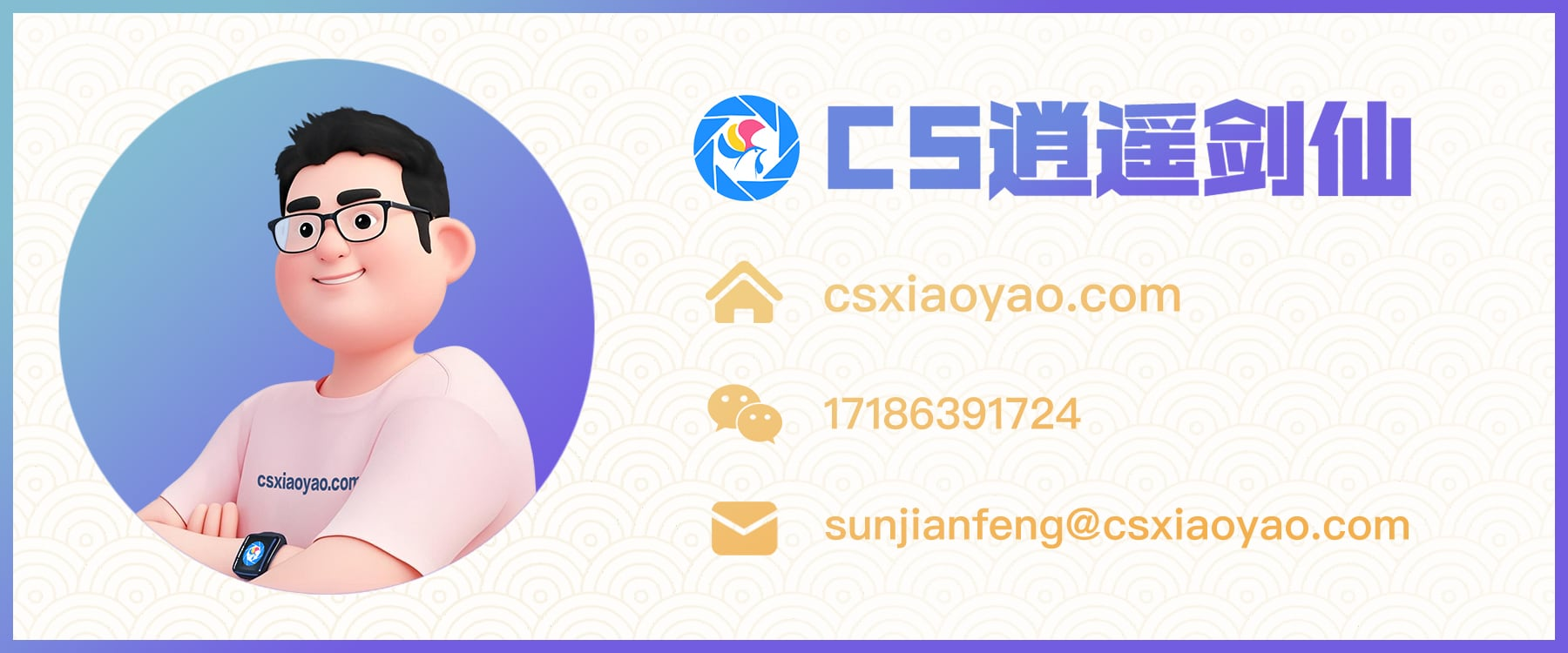# vue封装带提示框的单选/多选文本框组件

Write By CS逍遥剑仙

GitHub: github.com/csxiaoyaojianxian
Email: sunjianfeng@csxiaoyao.com
QQ: 1724338257

## 1. 组件化思想## 2. 接口参数设计

1. v-model (输入值，String，双向绑定)

1. size (尺寸，String， medium / small / mini)

2. opt (选项列表，Array，kv数组形如[{key:1, value:xxx}])

3. seperator (分隔符，String，如’,’、’|’、’-‘)

4. multiple (是否支持多选，Boolean)

5. placeholder (提示，String)

调用方式如下：

``````<cs-select
v-model="value" // value
size="mini" // 尺寸
:opt="optParams.kv" // 选项
seperator="," // 分隔符
:multiple="true">
</cs-select>
``````

## 3. 提示框显示隐藏交互实现

``````<template>
<div>
<!-- 输入框 -->
<el-input
@focus="onfocus
@blur="onblur>
</el-input>
<!-- 提示框 -->
<div v-if="show && opt.length > 0">
<el-row>
<el-col :span="8" v-for="(item, index) in opt" :key="index">
{{item.value}}
</el-col>
</el-row>
</div>
</div>
</template>
``````### 3.1 尝试方案1: click事件主动聚焦

``````clickEvent () {
this.show = true // 设置提示框显示
this.\$refs.input.\$el.querySelector('input').focus() // 设置主动聚焦
}
``````### 3.2 尝试方案2: blur事件添加延时器 + 开关变量

``````blurEvent () {
setTimeout(() => {
this.show = false
}, 200)
}
``````

``````focusEvent () {
this.show = true
this.canClose = true // 聚焦时打开开关
},
blurEvent () {
if (this.canClose) {
setTimeout(() => {
this.show = false // 只有开关打开时才执行关闭
}, 200)
}
},
clickEvent (key) {
this.canClose = false // 点击提示选项，关闭开关
this.show = true
...
}
``````

### 3.3 尝试方案3: 不使用blur，关闭方法改为事件委托

``````\$('body').on('click', (event) => {
this.show = false
})
\$('body').on('click', className, (event) => {
this.show = true
})
``````

``````let randId = Math.round(Math.random()*100000)
this.className = `cs-select-\${randId}`
// 单独处理父容器，在父容器上绑定关闭事件
...
``````

### 3.4 尝试方案4: onfocus + onblur + mousedown + 开关

``````focusEvent () {
this.show = true
this.canClose = true // 聚焦时打开开关
},
blurEvent () {
if (this.canClose) {
this.show = false // 只有开关打开时才执行关闭
}
},
mousedownEvent (key) {
this.canClose = false // 点击提示选项，关闭开关
this.show = true
this.\$refs.input.\$el.querySelector('input').focus()
...
}
``````### 3.5 实现方案

`\$nextTick`:

``````focusEvent () {
this.show = true
this.canClose = true // 聚焦时打开开关
},
blurEvent () {
if (this.canClose) {
this.show = false // 只有开关打开时才执行关闭
}
},
mousedownEvent (key) {
this.canClose = false // 点击提示选项，关闭开关
this.show = true
this.\$nextTick(() => {
this.\$refs.input.\$el.querySelector('input').focus()
})
...
}
``````## 4. 组件数据双向绑定的实现

### 4.1 父子组件数据双向绑定

``````watch: {
inputVal: {
handler () {
...
let selectArray = this.inputFilter()
this.inputVal = selectArray.join(this.seperator)
// 同步数据
this.\$emit('update:value', this.inputVal)
},
immediate: true
}
}
``````

``````<cs-select
:value.sync="cond.value"
...>
</cs-select>
``````

``````model: {
prop: 'value',
event: 'change'
},
props: {
value: String
},
watch: {
inputVal: {
handler () {
let selectArray = this.inputFilter()
this.inputVal = selectArray.join(this.seperator)
// 同步数据
this.\$emit('change', this.inputVal)
},
immediate: true
}
}
``````

`v-model`方式进行双向数据绑定对开发者更友好，因此更加推荐。

### 4.2 输入值与选中状态双向绑定``````watch: {
inputVal: {
handler () {
// 更新选中状态
this.updateActive()
...
},
immediate: true
}
},
methods: {
select (item) {
...
// 处理选择的数据，区分单选和多选
// let reg = new RegExp(`(^\${item.key})|(,\${item.key}\$)|(,\${item.key},)`, 'g')
if (this.multiple === true) {
// 多选
if (this.active[item.key]) {
// 取消
if (index >= 0) {
selectArray.splice(index, 1)
}
} else {
// 选中
if (index < 0) {
selectArray.push(item.key)
}
}
} else {
// 单选
selectArray = [item.key]
}
// 更新输入值
this.inputVal = selectArray.join(this.seperator)
},
// 根据input更新选中状态
updateActive () {
...
}
}
``````

## 5. 组件应用与改进1. 目前的提示框的显示隐藏是通过监听mousedown等事件，很明显不能兼容移动端，可以考虑添加touch相关事件；
2. 在css布局方面没有判断用户可见的友好性，在极端情况下可能会超出屏幕范围；
3. 还不支持slot插槽和动态class属性等。【By CS逍遥剑仙】 未经允许不得转载：禅林阆苑 » vue封装带提示框的单选/多选文本框组件 【原创】

### 如果觉得有用就请我喝一杯咖啡吧

#### 支付宝扫一扫打赏#### 微信扫一扫打赏